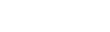# Vectors are somewhat similar to arrays but somewhat different too. Sounds confusing? Don't worry. After reading this article, you will get a clear idea of vectors.Ayush

4 months ago | 2 min read
Follow# What is a Vector?

In the previous article, we looked at arrays and its basics. Vectors are somewhat similar to arrays but somewhat different too. Sounds confusing? Don't worry. After reading this article, you will get a clear idea of vectors.

## Similarities between Array and Vector

• Just like Array, Vector is a linear data structure that is used to store elements of the same data type.
• You can access an element via the operator function [], in both vectors and arrays.

# Differences between Array and Vector

• Vectors are also known as dynamic arrays. Now, what is a dynamic array?
• Dynamic array is a data structure whose size may change over time. Items are not only added or removed, but memory used changes, too.
• Arrays are static whereas Vectors are dynamic.
• Vectors occupy more memory than arrays since they are dynamic in nature.
• Arrays are index-based data structures but vectors are not index-based.
• Arrays are available in C & C++, but Vectors are only available in C++.

## Advantages of Vector over Arrays

• Arrays have to be deallocated explicitly if defined dynamically whereas vectors are automatically de-allocated from heap memory. Example:
``#include <bits/stdc++.h>using namespace std;int main(){    int* arr = new int; // Dynamic Implementation    delete[] arr; // array Explicitly deallocated    vector<int> v; // Automatic deallocation when variable goes out of scope    return 0;}``
• Arrays cannot be copied or assigned directly whereas Vectors can be copied or assigned directly.
• When array becomes full and new elements are inserted; no reallocation is done implicitly whereas When vector becomes larger than its capacity, reallocation is done implicitly.

# Syntax and In-Built Functions of a Vector.

This is how you can initialize a vector in C++ (Multiple Ways).

``// vector<data_type> name of vector;    vector<int> vector1;    vector<int>vector2(2) (initializes with size 2)    vector<int>vector3(2,0) (initializes with size 2 and sets all elements to 0)    vector<int>vector4 = {1,2,3,5}``

Here is a list of most commonly used in-built functions of a vector.

• vector.size() [To get the size of a vector]
• vector.sort() [To sort the vector]
• vector.push_back() [to add an element in the vector]
• vector.pop_back() [to remove the last element]
• vector.reverse() [to reverse the vector]
• vector.begin() [returns an iterator(pointer) to the start of the vector]
• vector.insert(vector.begin()+2,5) [Inserts 5 at the 2rd index in the vector]

What does these functions do? We will see it in the code snippet below.

# General Time Complexities.

• Pushing an element in the vector: O(1)
• Popping an element from the vector: O(1)
• Accessing an element: O(N)
• Pushing at a particular index: O(N)
• Deleting from a particular index: O(N)

## Code Snippet

``// TC is Time Complexity. I am writing the worst case Time Complexities.#include<bits/stdc++.h>using namespace std;int main(){int n;cin>>n;vectorvec1; // initializing a vectorfor(int i=0;i<n;i++){int x;cin>>x;vec1.push_back(x);}vec1.push_back(1);   // TC: O(1)vec1.pop_back(); // TC: O(1)vec1.insert(vec1.begin()+2,5); // O(N)sort(vec1.begin(),vec1.end()); // TC: O(N*Log(N))reverse(vec1.begin(),vec1.end());  // TC: O(N)cout<<"size is: "<<vec1.size()<<endl;  // to get the size of the vectorcout<<"Second element is: "<<vec1<<endl;  //}``

# Conclusion.

So this was all about the basics of vectors. We have discussed all the basic functions of the vector and its time complexities. Want to learn about advanced concepts of vector? Have a look at our next article.

UpvoteCreated by

Ayush

Follow

CS @ IIITG | Flutter Dev | Documenting my JourneyPost

Upvote

Downvote

Comment

Bookmark

Share

Related Articles